When you think you have an answer to one of the puzzles below, e-mail it to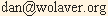and we'll e-mail you back the solution.

DARMO’S PUZZLE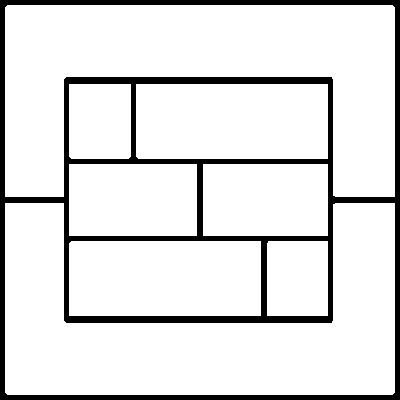Number the eight areas with 1 through 8 so that consecutive numbers are not adjacent.

LEIBNIZ’S PUZZLE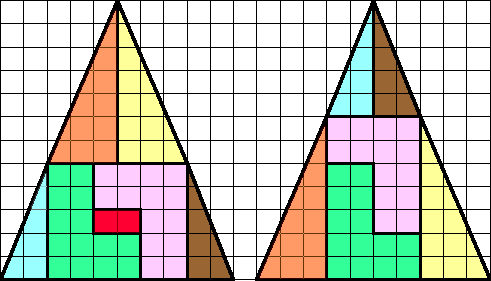Of the seven pieces on the left, discard one, and rearrange the remaining
six in the pattern shown on the right. Is there anything wrong here?

ACE'S PUZZLE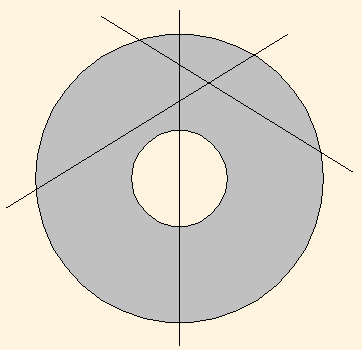With three straight cuts, divide a washer into nine pieces.
Leave the pieces in place after each cut.
(The cuts above form only seven pieces.)

DUNCAN’S PUZZLE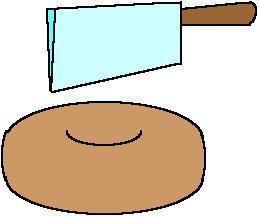With three straight cuts (they can be at an angle),
divide a doughnut into thirteen pieces.
Leave the pieces in place after each cut.

MY SISTER’S PUZZLE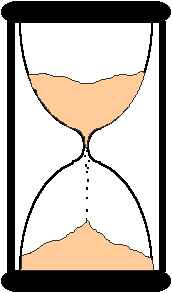My sister is three times as old as I was ten years ago, and she’s twice as old
as I was when she was my present age. How old is my sister?  How old am I?
Check to see if she's now three times my age ten years ago.
How old was I when she was my age now?  Is she twice that?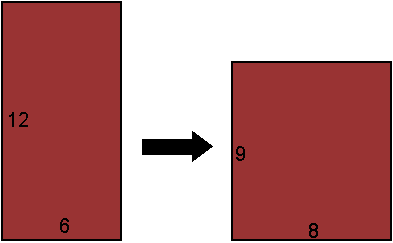Cut a 6 ´ 12 carpet into two pieces so they can be sewn together into an 8 ´ 9 carpet.

HOLZHAUER’S PUZZLE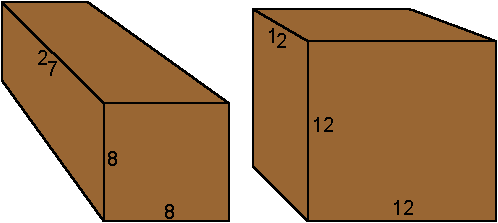Cut an 8 ´ 8 ´ 27-inch wood beam into four pieces
so they can be reassembled into a 12-inch cube.

TOM & RAY’S PUZZLE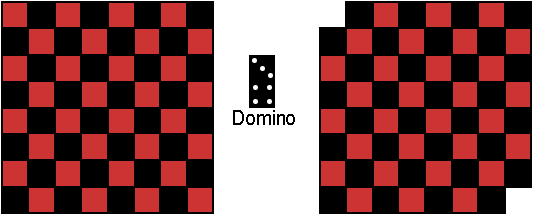Can you cover the checkerboard with 32 dominoes? Can you
cover the truncated checkerboard with 31 dominoes?

SHEARSON’S PUZZLE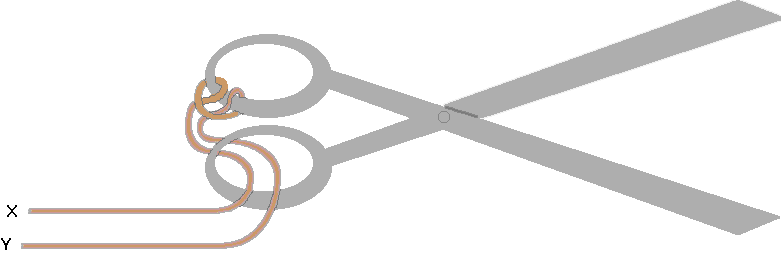Intertwine a cord with a pair of scissors as shown. With
ends X and Y anchored, remove the cord from the scissors.

LOUIS’S PUZZLE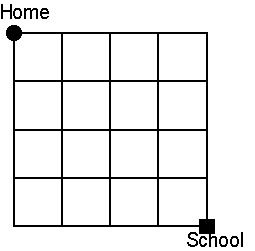Louis lives four blocks north and four blocks west of school. How many
different routes can he take to school (always heading south or east)?

MATT'S PUZZLE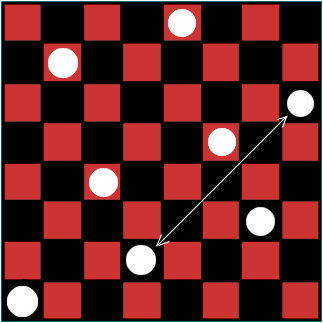Arrange eight queens on the chess board so no queen is
attacking another. The arrangement shown above almost
works; two queens still attack each other diagonally.

TERRY’S PUZZLE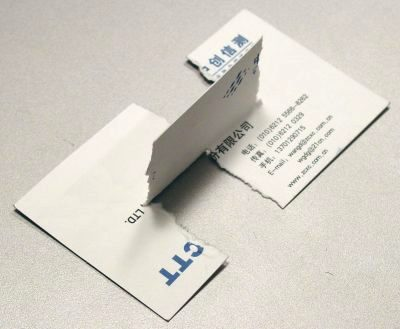RUSS’S PUZZLE

 For a sequence of three 1s and 0s, there are eight possible patterns: 000 001 010 011 100 101 110 111 For a sequence of n 1s and 0s, there are 2n possible patterns. For a sequence of three 1s and 0s with no adjacent 1s, there are five possible patterns: 000 001 010 100 101 For a sequence of n 1s and 0s with no adjacent 1s, how many possible patterns are there? (Try for a few values of n, see a pattern, and generalize. Then prove.)

ARNIE’S PUZZLE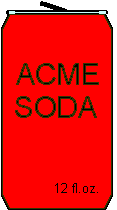Arnie has opened a 12-oz can of soda in the car. (The can itself weighs 1/2 oz.)
If he drinks nothing, the center of gravity is at the center of the can. If he drinks
it all, the center of gravity is again at the center of the can. How much soda should
Arnie drink so the can has the least chance of tipping over if he comes to a sudden
stop? (Assume a fluid ounce weighs an ounce.)

HOWARD’S PUZZLEA stick is broken at random in two places. What
are the odds that you can form a triangle of the pieces?

LIBRO’S PUZZLE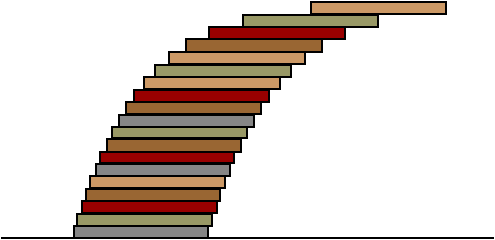What is the minimum number of books in a skewed stack so the
top book projects one book width beyond the bottom book?
How many so it projects two book widths?

BINNY'S PUZZLE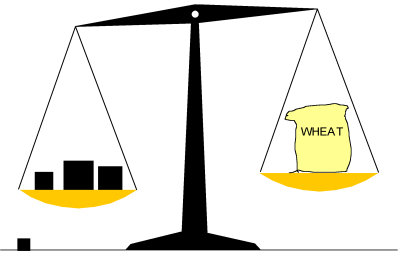Pick four weights so some combination of them in the left pan
will balance a sack of wheat from 1 to 15 lb. by 1-lb. increments.

TRYON'S PUZZLE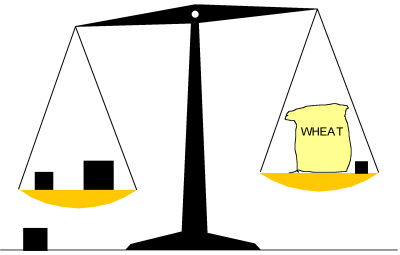Pick four weights so some combination of them in the left and right
pans will balance a sack of wheat from 1 to 40 lb. by 1-lb. increments.

JIMMY'S PUZZLE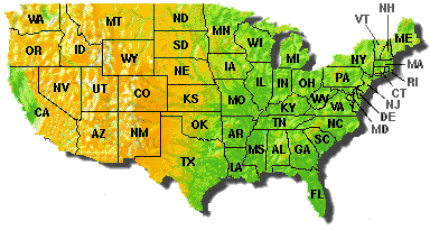Jimmy, in a west-coast state, calls his mother in an east-coast state. After a while she says she really should be getting to bed, and mentions the time. "Hey!" says Jimmy, "it's the same time here!" What states are they in? (There is no trick in the wording here. A "west-coast state" is Washington, Oregon, or California, and an "east-coast state" is one from Maine to Florida. "The same time" means what the clock reads in that time zone when correctly set to standard or daylight-saving time, according to the time of year.)

(USA time zones)

GRENZA'S PUZZLE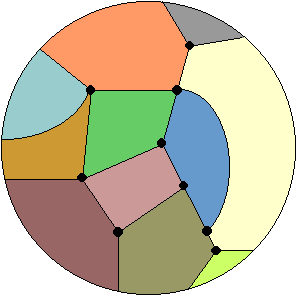A planet with no bodies of water is divided into countries by lines that meet (terminate) at points. No country is an "island" (surrounded by one country). There are 37 lines and 15 points. How many countries are there?

PIERRE'S PUZZLE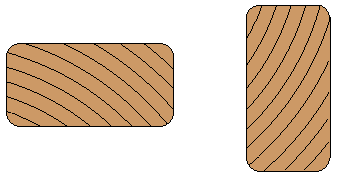Pierre has thrown several pieces of 2×4 lumber into the lake. Some pieces float with the 4" side up and some with the 2" side up. What is the deciding factor?

RACHEL'S PUZZLE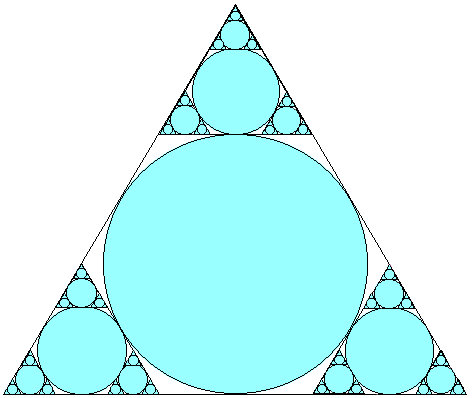There is an infinite number of circles. What fraction
of the area within the triangle do the circles occupy?

DAN’S PUZZLE

x y = y x

 For a given real x greater than one, the equation almost always has two solutions for y. For example, for x = 2, then y = 2 and y = 4 are both solutions. For x = 5.3, then y = 5.3 and y = 1.71586398526979... are both solutions. But there is a real x greater than one for which there is only one solution for y.

GREG'S PUZZLE

Bisect a line segment using only a compass (no straight edge).

MARTIN'S PUZZLE

 Disprove the assertion that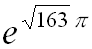is an integer.

CUBBY'S PUZZLE

 Prove the assertion thatis an integer.

PETTIFERM'S PUZZLE

 It seems that 2p–1 – 1 is always divisible by p if p is prime. For example, for p = 5, then 25–1 – 1 = 24 – 1 = 15, which is divisible by 5. For p = 7, then 27–1 – 1 = 26 – 1 = 63, which is divisible by 7. Prove that 2p–1 – 1 is, in fact, divisible by p if p is prime. Find a p that is not prime for which 2p–1 – 1 is divisible by p.